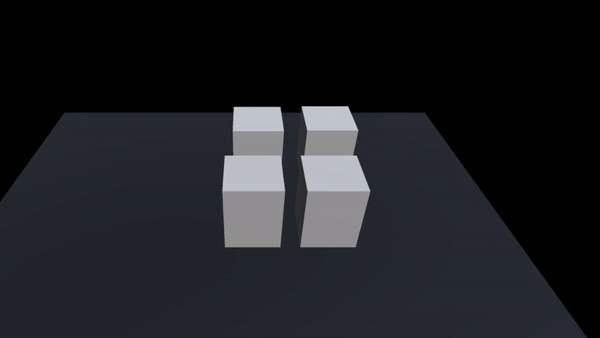# Scale object by perlinnoise randomized.

I would like to make a couple of 3d objects scale according to a Perlin noise. But their movements isnt as random as I would like it to be. When I press play they scale random once and then they follow the same routes. I’ve searched YouTube for the answer and this I what I’ve tried so far:

`public float perlinNoise = 0f;
public float multiplier = 0f;

``````private void Update()
{
perlinNoise = Mathf.PerlinNoise(Time.time, 0);
transform.localScale = new Vector3(1f, perlinNoise * multiplier + 1f, 1f);
}`
``````

Here’s a how it looks like:All the objects use the same values for the PerlinNoise method, so it’s normal they scale the same.

``````private float perlinNoise = 0f;
public float amplitude = 1f;
public float multiplier = 1f;
private void Update()
{
perlinNoise = Mathf.PerlinNoise(transform.position.x * multiplier + Time.time, transform.position.z * multiplier);
transform.localScale = new Vector3(1f, perlinNoise * amplitude + 1f, 1f);
}
``````